## RS Aggarwal Class 8 Solutions Chapter 1 Rational Numbers Ex 1G

These Solutions are part of RS Aggarwal Solutions Class 8. Here we have given RS Aggarwal Solutions Class 8 Chapter 1 Rational Numbers Ex 1G.

Other Exercises

Question 1.
Solution:
Total length of rope = 11 m.
Sum of lengths of two parts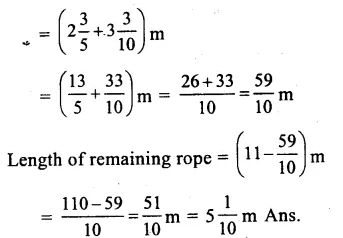Question 2.
Solution:
Total weight of rice and drum
= $$40\frac { 1 }{ 6 }$$ kg
= $$13\frac { 3 }{ 4 }$$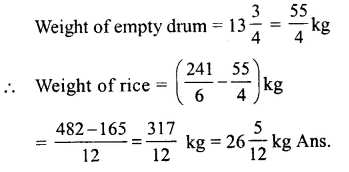Question 3.
Solution:
Total weight of three types of fruits
= $$19\frac { 1 }{ 3 }$$ kg
= $$\\ \frac { 58 }{ 3 }$$ kgQuestion 4.
Solution:
Total earnings = Rs 160Question 5.
Solution:
Cost of 1m cloth = Rs $$63\frac { 3 }{ 4 }$$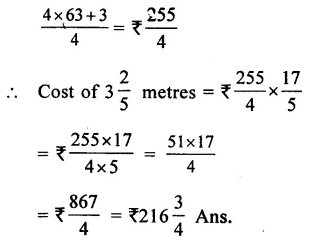Question 6.
Solution:
Distance covered in 1 hour
= $$60\frac { 2 }{ 5 }$$
= $$\\ \frac { 302 }{ 5 }$$Question 7.
Solution:
Length of rectangular park
= $$36\frac { 3 }{ 5 }$$ m
= $$\\ \frac { 183 }{ 5 }$$
and breadth = $$16\frac { 2 }{ 3 }$$ = $$\\ \frac { 50 }{ 3 }$$ m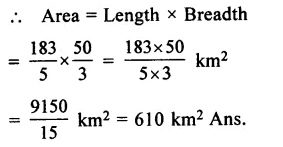Question 8.
Solution:
Side of a square plot = $$8\frac { 1 }{ 2 }$$ m
= $$\\ \frac { 17 }{ 2 }$$ m
Area = (Side)² = Side x Side
= $$\\ \frac { 17 }{ 2 }$$ x $$\\ \frac { 17 }{ 2 }$$ m²
= $$\\ \frac { 289 }{ 4 }$$ m²
= $$72\frac { 1 }{ 4 }$$ m²

Question 9.
Solution:
Cost of 1 litre petrol = Rs $$63\frac { 3 }{ 4 }$$
= Rs $$\\ \frac { 255 }{ 4 }$$
Cost of 34 litres of petrol
= $$\\ \frac { 255 }{ 4 }$$ x 34
= $$\\ \frac { 255X17 }{ 2 }$$
= $$\\ \frac { 4335 }{ 2 }$$
= Rs $$2167\frac { 1 }{ 2 }$$

Question 10.
Solution:
Distance covered in 1 hour = 1020 km.
Distance covered in $$4\frac { 1 }{ 6 }$$ hours
= 1020 x $$4\frac { 1 }{ 6 }$$
= 1020 x $$\\ \frac { 25 }{ 6 }$$ km
= $$\\ \frac { 25500 }{ 6 }$$
= 4250 km. Ans.

Question 11.
Solution:
Cost of $$3\frac { 1 }{ 2 }$$ metres cloth
= Rs $$166\frac { 1 }{ 4 }$$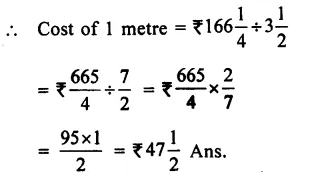Question 12.
Solution:
Total length of piece of chord
= $$71\frac { 1 }{ 2 }$$
No. of pieces = 26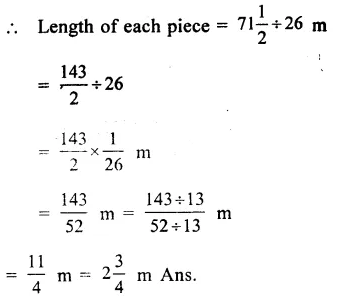Question 13.
Solution:
Area of a room = $$65\frac { 1 }{ 4 }$$ m²
Breadth = $$5\frac { 7 }{ 16 }$$ m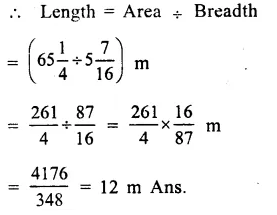Question 14.
Solution:
Product of two fractions = $$9\frac { 3 }{ 5 }$$
= $$\\ \frac { 48 }{ 5 }$$
One fraction = $$9\frac { 3 }{ 7 }$$ = $$\\ \frac { 66 }{ 7 }$$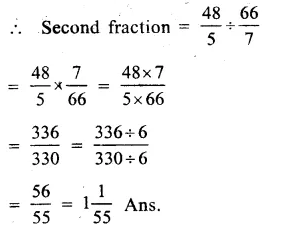Question 15.
Solution:
Let total number of students =1
and no.of boys = $$\\ \frac { 5 }{ 8 }$$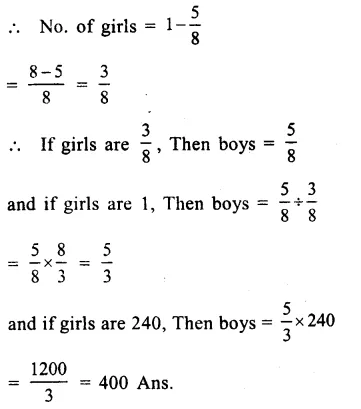Question 16.
Solution:
Let no of pages = 1
Then no. of pages read = $$\\ \frac { 7 }{ 9 }$$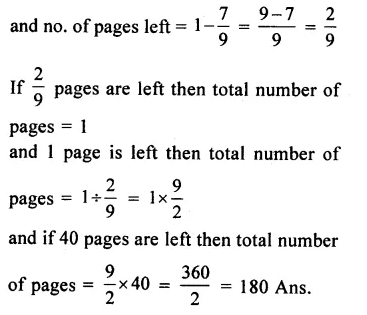Question 17.
Solution:
Total amount, Rita has = Rs 300
Amount spent on notebooks = $$\\ \frac { 1 }{ 3 }$$ of 300Question 18.
Solution:
Total amount earned by Amit = Rs 32000
Amount spent on food = $$\\ \frac { 1 }{ 4 }$$ of Rs 32000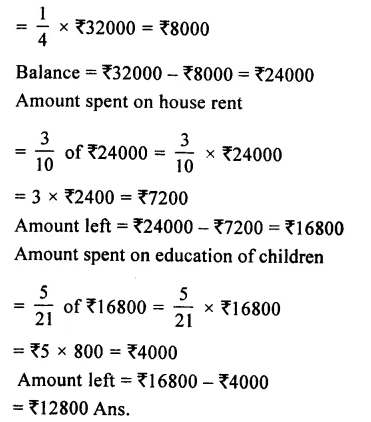Question 19.
Solution:
Let number = 1
Then difference between $$\\ \frac { 3 }{ 5 }$$ and $$\\ \frac { 2 }{ 7 }$$Question 20.
Solution:
Let total number of spectators = 1
No. of spectators in covered place = $$\\ \frac { 2 }{ 7 }$$ of
1 = $$\\ \frac { 2 }{ 7 }$$Hope given RS Aggarwal Solutions Class 8 Chapter 1 Rational Numbers Ex 1G are helpful to complete your math homework.

If you have any doubts, please comment below. Learn Insta try to provide online math tutoring for you.